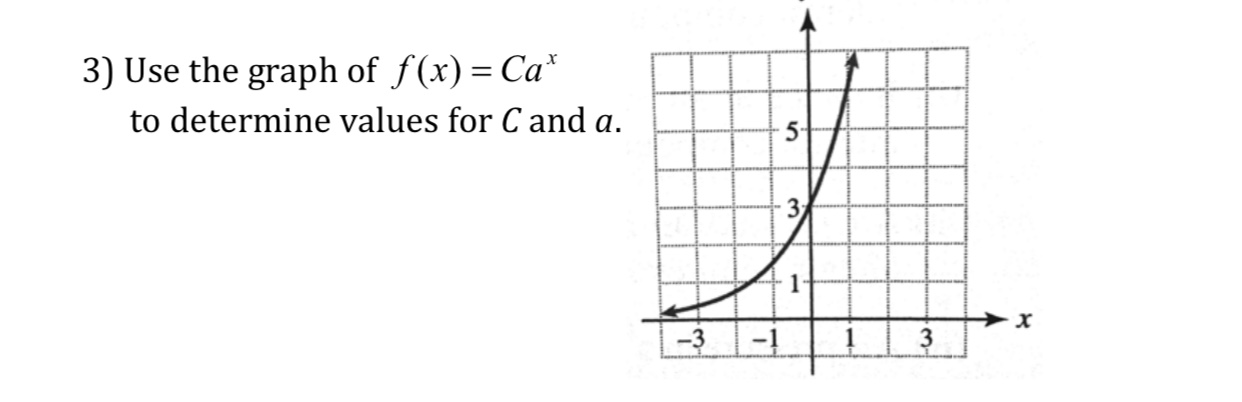3) Use the graph of f(x)-Сахto determine values for C and a-3 3

Questionhelp_outlineImage Transcriptionclose3) Use the graph of f(x)-Сах to determine values for C and a -3 3 fullscreen
Step 1

It is clear from graph that when x=0 , f(x)=3 , that is f(0)=3, using this , we can calculate C.

Step 2

Also, when x=1 , f(x)=6 , that is f(1)=6,...

Want to see the full answer?

See Solution

Want to see this answer and more?

Our solutions are written by experts, many with advanced degrees, and available 24/7

See Solution
Tagged in

Other